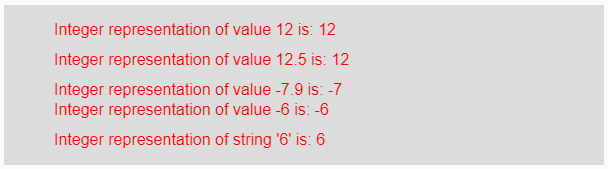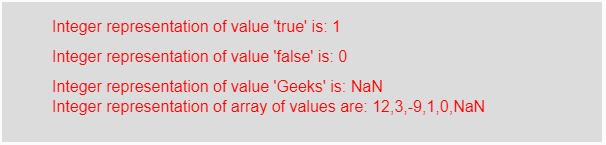Related Articles
p5.js | int() function
• Last Updated : 11 Feb, 2021

The int() function in p5.js is used to convert the given boolean, integer, and float value into its integer representation.

Syntax:

`int(ns)`

or

`int(n, radix)`

Parameters: This function accepts three types of the parameter which are listed below:

• n: It stores the value which needs to converts into integer. The possible value of n is string, boolean, or number.
• radix: It stores the radix part to which the number is converted.
• ns: It stores different types of elements into the array.

Return Value: It returns the converted integer representation.
Below programs illustrate the int() function in p5.js:
Example 1: This example uses int() function to convert input value into its integer value.

## javascript

 `function` `setup() {`` ` `    ``// Creating Canvas size``    ``createCanvas(600, 160);``}`` ` `function` `draw() {``     ` `    ``// Set the background color``    ``background(220);``   ` `    ``// Initializing some strings``    ``let Value1 = 12;``    ``let Value2 = 12.5;``    ``let Value3 = -7.9;``    ``let Value4 = -6;``    ``let Value5 = ``"6"``;``   ` `    ``// Calling to int() function.``    ``let A = int(Value1);``    ``let B = int(Value2);``    ``let C = int(Value3);``    ``let D = int(Value4);``    ``let E = int(Value5);``     ` `    ``// Set the size of text``    ``textSize(16);``     ` `    ``// Set the text color``    ``fill(color(``'red'``));``   ` `    ``// Getting integer representation``    ``text(``"Integer representation of value 12 is: "` `+ A, 50, 30);``    ``text(``"Integer representation of value 12.5 is: "` `+ B, 50, 60);``    ``text(``"Integer representation of value -7.9 is: "` `+ C, 50, 90);``    ``text(``"Integer representation of value -6 is: "` `+ D, 50, 110);``    ``text(``"Integer representation of string '6' is: "` `+ E, 50, 140);``} `

Output:Example 2: This example uses int() function to convert input value into its integer value.

## javascript

 `function` `setup() {`` ` `    ``// Creating Canvas size``    ``createCanvas(600, 140);``}`` ` `function` `draw() {``     ` `    ``// Set the background color``    ``background(220);``   ` `    ``// Initializing some values``    ``let Value1 = ``true``;``    ``let Value2 = ``false``;``    ``let Value3 = ``"Geeks"``;``    ``let Value4 = [12, 3.6, -9.8, ``true``, ``false``, ``"Geeks"``];``   ` `    ``// Calling to int() function.``    ``let A = int(Value1);``    ``let B = int(Value2);``    ``let C = int(Value3);``    ``let D = int(Value4);``     ` `    ``// Set the size of text``    ``textSize(16);``     ` `    ``// Set the text color``    ``fill(color(``'red'``));``   ` `    ``// Getting integer representation``    ``text(``"Integer representation of value 'true' is: "` `+ A, 50, 30);``    ``text(``"Integer representation of value 'false' is: "` `+ B, 50, 60);``    ``text(``"Integer representation of value 'Geeks' is: "` `+ C, 50, 90);``    ``text(``"Integer representation of array of values are: "` `+ D, 50, 110);``} `

Output:Note: From the above program, if the value of the parameter is number then it returns the output as integer representation, for false it returns 0 and for true it returns 1 and for string values, it returns NaN i.e, not a number.

Reference: https://p5js.org/reference/#/p5/int

Attention reader! Don’t stop learning now. Get hold of all the important DSA concepts with the DSA Self Paced Course at a student-friendly price and become industry ready.

My Personal Notes arrow_drop_up